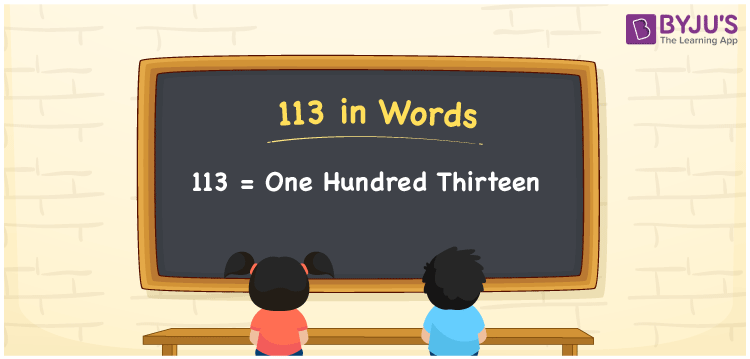# 113 in Words

113 in words is written as One Hundred Thirteen. The name of number 113 in English is “One Hundred Thirteen”. The word One Hundred Thirteen is used in many real-life situations. For example, there are One Hundred Thirteen students in a classroom. Thus, it is a counting number. Also, to represent the currency equal to 113, we can write it in words as Rupees One Hundred Thirteen or One Hundred Thirteen rupees. Therefore, it is necessary to learn the numbers in words, for the ease of understanding and expressing them.

 113 in words One Hundred Thirteen One Hundred Thirteen in Numbers 113

## 113 in English Words## How to Write 113 in Words?

If we know the place value of digits of 113, then we can easily express it in words. The place value is basically the position of a digit in a number. 113 is a three-digit number, therefore, we can specify the position of each digit of 113 in a place value chart. In the Indian numbering system, the order of place value of digits from right to left is given by:

 Hundreds Tens Ones 1 1 3

We can write it in expanded form as:

1 × Hundred + 1 × Ten + 3 × One

= 1 × 100 + 1 × 10 + 3 × 1

= 100 + 10 + 3

= 113

= One Hundred Thirteen

Therefore, 113 in words is written as One Hundred Thirteens.

113 is a natural number that precedes 114 and succeeds 112.

113 in words – One Hundred Thirteen

Is 113 an odd number? – Yes

Is 113 an even number? – No

Is 113 a perfect square number? – No

Is 113 a perfect cube number? – No

Is 113 a prime number? – No

Is 113 a composite number? – Yes

## Frequently Asked Questions on 113 in Words

### How to write 113 in words?

113 in English is written as “One Hundred Thirteen”.

### Is 113 an odd number or even number?

113 is an odd number because it is not wholly divisible by 2.

### Write One Hundred Thirteen in numbers.

One Hundred Thirteen in numbers is 113.